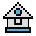Home

# WINDOW Statement

#### Purpose:

To draw lines, graphics, and objects in space not bounded by the physical аlimits of the screen.

#### Syntax:

`WINDOW[[SCREEN](x1,y1)-(x2,y2)]`

(x1,y1), (x2,y2) are the coordinates defined by the user. These coordinates, called the world coordinates, may be any single-precision, floating-point number. They define the world coordinate space that graphics statements map into the physical coordinate space, as defined by the VIEW statement.

WINDOW is the rectangular region in the world coordinate space. It allows zoom and pan. It allows the user to draw lines, graphics, and objects in space not bounded by the physical limits of the screen. To do this the user specifies the world coordinate pairs (x1,y1) and (x2,y2.) GW-BASIC then converts the world coordinate pairs into the appropriate physical coordinate pairs for subsequent display within screen space.

Window inverts, with the screen attribute omitted, the y coordinate on subsequent graphics statements. This places the (x1,y1) coordinate in the lower-left and the (x2,y2) coordinate in the upper-right of the screen. This allows the screen to be viewed in true Cartesian coordinates.

The coordinates are not inverted when the SCREEN attribute is included. This places the (x1,y1) coordinate in the upper-left and the (x2,y2) coordinate in the lower-right corner of the screen.

The WINDOW statement sorts the x and y argument pairs into ascending order. For example:

`WINDOW (50, 50)-(10, 10)`
becomes
`WINDOW (10, 10)-(50, 50)`

Or

`WINDOW (-2, 2)-(2, -2)`
becomes
`WINDOW (-2, -2)-(2, 2)`

All coordinate pairs of x and y are valid, except that x1 cannot equal x2 and y1 cannot equal y2.

WINDOW with no arguments disables previous window statements.

#### Example 1:

If you type:

`NEW: SCREEN 2 `

the screen uses the standard coordinate attributes:

```(0, 0)аааааааааа (320, 0)аааааааа (639, 0)
а ↓ y increases
ааааааааааааааа (320, 100)

(0, 199) аааааа (320, 199)ааааа (639, 199)```

#### Example 2:

If you type:

`WINDOW (-1, -1)-(1, 1)`

the screen uses the Cartesian coordinates:

```(-1, 1) ааааааааа (0, 1)ааааааааааа (1, 1)
а ↑ y increases
ааааааааааааааааа (0, 0)
а ↓ y decreases
(-1, -1)ааааааааа (0, -1)аааааааааа (1, -1)```

#### Example 3:

If you type:

`WINDOW SCREEN (-1, -1)-(1, 1)`

the screen uses the non-inverted coordinate:

```(-1, -1)ааааааааа (0, -1)аааааааааа (1, -1)
↑ y decreases
ааааааааааааааааа (0, 0)
↓ y increases
(-1, 1)аааааааааа (0, 1)ааааааааааа (1, 1)```

RUN, SCREEN, and WINDOW with no attributes disable any WINDOW definitions and return the screen to its normal physical coordinates.